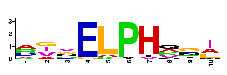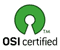Center for Bioinformatics and Computational Biology### Index

1. Introduction
2. Running ELPH
1. Running the program as a motif finder
2. Running the program to compare the significant difference of a motif appearances in two different files
3. Obtaining ELPH

### I. Introduction

ELPH is a general-purpose Gibbs sampler for finding motifs in a set of DNA or protein sequences. The program takes as input a set containing anywhere from a few dozen to thousands of sequences, and searches through them for the most common motif, assuming that each sequence contains one copy of the motif. We have used ELPH to find patterns such as ribosome binding sites (RBSs) and exon splicing enhancers (ESEs).

### II. Running ELPH

There are two different ways to run the program: as a motif finder, or as a tool to measure if there is any significant difference between the appearances of a motif in two different files.

### II.1 Running the program as a motif finder

In this case the input of ELPH is a file in multi FASTA format containing the sequences:
elph <multi-fasta_file> [options]
Common usage:
elph DNAseqs.fasta LEN=5

### II.1.1 Algorithm overview

The common usage of the program is to use as motif finder a Gibbs site sampler. The programs begins by randomly selecting one motif element in each sequence. After this initially setup the program iteratively runs through the following two steps:

- predictive update step: one sequence from the input file is selected, beginning with the first sequence and proceeding to the last sequence. The current motif element from the sequence is added too the background and the motif matrix is updated accordingly.

- sampling step: each possible starting position for a motif in the given sequence is assigned a probability of being a motif starting at that position; after that, a motif element is assigned to the sequence by performing a weighted sample from all the possible positions.

These steps are repeated until a local maximum is reached or a fixed maximum number of iterations are made. The Gibbs sampler is restarted several times with a different seed in order to avoid trappings into a local maximum. Once the motif alignment is found, the posteriori value of the alignment is computed. An optimizing procedure is run to maximize this posteriori value returning the MAP (maximum a posteriori probability) of the motif.

### II.1.2 Command line options

Several command line options can be specified:

• LEN=n : n is the length of the searched motif; if the length of the motif is not given, the program will ask for it from stdin.
• ITERNO=n : n represents the maximum number of times that the Gibbs sampler is restarted in order to avoid trapping into the local maximum; default=10.
• MAXLOOP=n : n represents the maximum number of iterations used by the program to compute the local maximum; default = 500.
• SGFNO=n : n is the number of iterations to compute significance of motif (see also the -g option); default = 1000.
• -h : prints a help with the options the program.
• -o <out_file> : write output in <out_file> instead of stdout
• -a : by default the multiFASTA file is considered to contain DNA sequences; if this option is specified the input file would be considered to contain amino acid sequences (this option has not been tested yet!).
• -s <seed> : sets the seed for the random generation.
• -p n : n represents the number of iterations before deciding that the local maximum has been reached; default=20.
• -b : if this options is specified then only matrix frequencies for the background and the motif are printed; i.e. the positions of each motif element within the sequence are not shown.
• -x : normally the output of the program shows the motif elements that contributed to the computation of the motif matrix; if the -x option is used the output will also show for each sequence those positions which give the maximal score by using the computed motif matrix (can be different from the motif elements' positions).
• -m <motif> : use the given pattern <motif> to compute its best fit matrix to the data.
• -g : if this option is specified then a significance of the motif found is computed by comparing the appearances of the motif elements within the input file to the appearances of the motif within a randomly generated file containing sequences of the same lengths as in the input file and with the same residue distribution. The randomly generated file is paired to the input file. Given the motif matrix, motif sampling is performed a number of times (specified by SGFNO), and a probability of occurrence of each motif element is computed in the two paired samples. Two significance tests are used: the Wilcoxon pair test (most reliable) and the student test.
• -d : this option regards the way the significance of the motif is computed; when -v is specified, the probability of occurrence of each motif element is estimated from the motif matrix, so no there isn't necessary to run the Gibbs sampler SGFNO times; this option should accompany the -g option.
• -v : if this option is given then the Gibbs sampler is not used anymore, and the motif is computed in a deterministic way which maximizes the MAP (faster).
• -e : only when an additional file is used to test the significance of the motif: find only the motifs that exactly match the input pattern (-m or -t options)
• -n [0..5] : degree of Markov chain used to generate the random file used to test the significance of the motif default = 2
• -l : if the -m option is specified too, computes the Least Likely Consensus (LLC) score for the given motif; this score measure the information content of the motif combined in respect to its background rareness.

### II.2 Running the program to compare the significant difference of a motif appearances in two different files

The input to ELPH in this case consists of two files in multi FASTA format:

elph <multi-fasta_file-1> <multi-fasta_file-2> [options]

The program computes a motif in <multi-fasta_file-1 > and then estimates if the motif is significantly more represented in <multi-fasta_file-1> compared to <multi-fasta_file-2>. All the options for computing the motif can be specified. There is an additional option which can only be given to this way of running the program:

-t <matrix> : test if there is significant difference between the two input files for a given motif matrix;  <matrix> is the file containing the motif matrix

### III. Obtaining ELPH

ELPH is available free of charge under the open-source Artistic License.